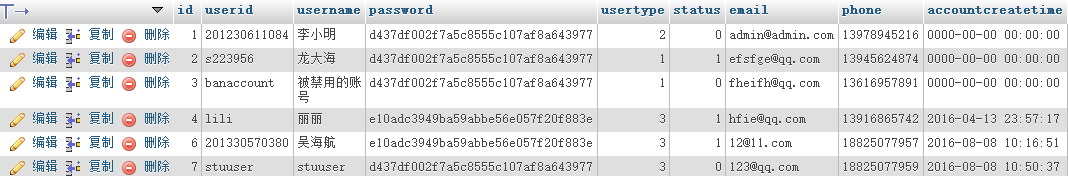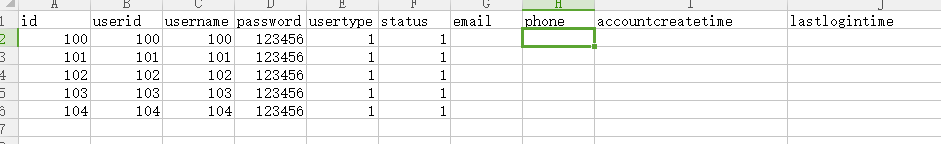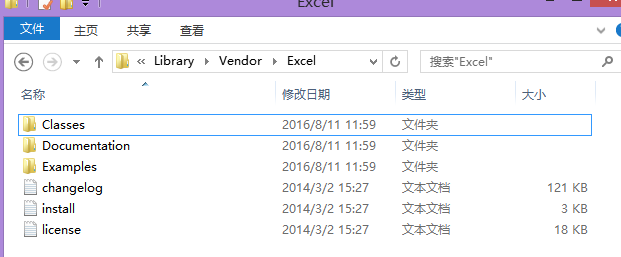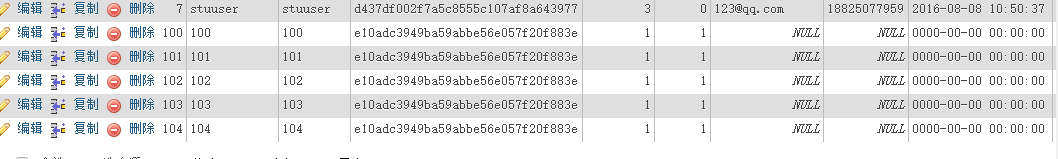# 如何使用PHPEXCEL导入EXCEL文件

1.我的数据表结构是这样的（。。。有没有同学不知道怎么看数据库的，反正我一开始连这个都不知道…这个是右击wampserver，就会看见一个PHPadmin,点就可以了）

2.要导入的EXCEL文件是这样的1.下载PHPExcel,这是待会儿我们处理EXCEL文件的第三方类库，并且将下载好的文件复制到这个路径下面wamp\www\ThinkPHP\Library\Vendor1.根据MVC模式，我们也来分别弄一个控制器（ExcelController.class.php），视图(excel.html)和模型(UsersModel)。

*a.一般控制器的名字要和对应视图名字一样（去掉个Controller），控制器文件的后缀一定是.class.php。
b.模型的名字和对应数据表的名字是一样的，当然要弄个前缀后缀什么的，比如，我的模型名字是UsersModel，那么它操纵的数据表就是think_users.*

2.控制器代码

<?php
namespace Home\Controller;
use Think\Controller;

Vendor('Excel.Classes.PHPExcel');/*引入PHPExcel类。我就是在这一步卡了超级久，总是报错找不到这个类，

class ExcelController extends CommonController {
//这个方法就是显示出对应的html页面，因为这个叫excelcontroller,所以它就会显示excel.html。
public function excel()
{
$this->display(); } /* 这个函数就是最重要的函数啦，用来读取EXCEL文件，然后将它添加到数据库中，下面会有详细的解释*/ public function add() {/*下面三行是取得由页面传过来的excel文件，然后经过一些字符串处理，取得了文件名，用于判断是不是excel文件*/$tmp_file = $_FILES ['file_stu'] ['tmp_name'];$file_types = explode ( ".", $_FILES ['file_stu'] ['name'] );$file_type = $file_types [count ($file_types ) - 1];
/*判别是不是.xls文件，判别是不是excel文件*/
if (strtolower ( $file_type ) != "xlsx" && strtolower ($file_type ) != "xls")
{
$this->error ( '不是Excel文件，重新上传' ); } /*设置上传路径，就是上传的excel文件保存的地方，注意路径的分割是用的正斜杠不是反斜杠*/$savePath = 'E:/php/wamp/www/Public/';
/*以时间来命名上传的文件*/
$str = date ( 'Ymdhis' );$file_name = $str . "." .$file_type;

/
/*是否上传成功*/
if (!move_uploaded_file ( $_FILES ['file_stu'] ['tmp_name'],$savePath.$file_name )) {$this->error ( '上传失败' );
}

//特别注意这里PHPExel前面有一个反斜杠，\
//load里面是对应的excel文件，通过这个文件来生成PHPExcel
$objPHPExcel=\PHPExcel_IOFactory::load("E:/php/wamp/www/Public/".$file_name);
//获取sheet0，就是excel第一个sheet
$sheet =$objPHPExcel->getSheet(0);

$highestRow =$sheet->getHighestRow(); // 取得总行数

$highestColumn =$sheet->getHighestColumn(); // 取得总列数
//调用ThinkPHP中的M方法来实例化一个USERS模型，从而调用SQL操作
$User = M("Users"); //这个循环是一行一行的把excel里面的值取出来，存到$data数组里面
for($j=2;$j<=$highestRow;$j++)
{
$data['id']=$objPHPExcel->getActiveSheet()->getCell("A".$j)->getValue();//获取A列的值$data['userid']= $objPHPExcel->getActiveSheet()->getCell("B".$j)->getValue();
$data['username']=$objPHPExcel->getActiveSheet()->getCell("C".$j)->getValue();$data['password']= md5($objPHPExcel->getActiveSheet()->getCell("D".$j)->getValue());
$data['usertype']=$objPHPExcel->getActiveSheet()->getCell("E".$j)->getValue();$data['status']= $objPHPExcel->getActiveSheet()->getCell("F".$j)->getValue();
//调用add操作，将数据存入数据库
$User->add($data);
}
}
}


3.excel.html文件

 <!-- 需要注意的是action属性的意思是，将表单数据传给action所指向的页面，
比如这里的action就是表示把表单数据（也就是excel文件）传给excelcontroller的add方法处理。 -->
<form method="post" action="{:U('Excel/add')}" enctype="multipart/form-data">
<h3>导入Excel表：</h3><input  type="file" name="file_stu" />
<input type="submit"  value="导入" />
</form>

4.UsersModel文件（这个不重要，随便写点都行）

<?php
namespace Home\Model;
use Think\Model;

/**
*
*/
class UsersModel extends Model
{

protected $_validate = array( array('userid', '', '用户名（学号）不能为空！', 0, 'unique', 1), //默认情况下用正则进行验证 array('username', 'require', '请输入你的真实姓名', 1), // 在新增的时候验证name字段是否唯一 array('usertype', 'require', '请输入你的账号类型'), array('email', '', '该邮箱已被占用', 0, 'unique', 1), // 新增的时候email字段是否唯一 array('phone', '', '该手机号码已被占用', 0, 'unique', 1), // 新增的时候mobile字段是否唯一 // 正则验证密码 [需包含字母数字以及@*#中的一种,长度为6-22位] array('password', '/^([a-zA-Z0-9@*#]{32})$/', '密码格式不正确,请重新输入！', 0),
array('repassword', 'password', '确认密码不正确', 0, 'confirm'), // 验证确认密码是否和密码一致
array('email', 'email', '邮箱格式不正确'), // 内置正则验证邮箱格式
array('phone', '/^1\d{9}\$/', '手机号码格式不正确', 0), // 正则表达式验证手机号码

}